ISSN: 1070-4698

# Vol. 42

Front:[PDF file] Back:[PDF file]
Latest Volume
All Volumes
All Issues

PIER Vol. 42, 303-337, 2003. doi:10.2528/PIER03020302

###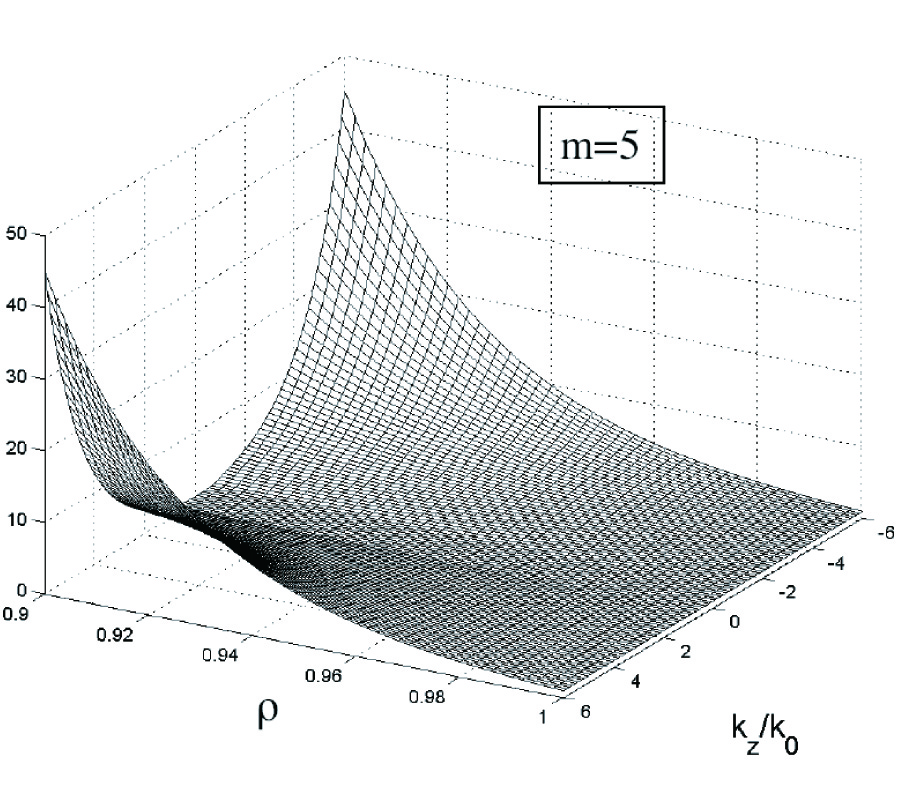Electromagnetic Holography on Cylindrical Surfaces Using k -Space Transformations

Spectral decomposition in 2-D kz-m space is used to develop transfer functions that relate modal electromagnetic fields on concentric cylindrical surfaces. It is shown that all time-average radiated power is generated by superluminal modes (phase velocity vz > c) which are confined to the baseband |kz| > k0. Subluminal modes, with kz outside of the radiated band, are radially evanescent but permit recovery of imaging resolution that exceeds the usual diffraction limit provided by the radiated fields. Outward translation between cylinder surfaces is found to have a stable low-pass 2-D transfer characteristic in kz-m space, where spatial resolution decreases with increased radius. The inverse transfer functions for inward translation of field components (termed backpropagation) employ a high-pass process that amplifies subluminal evanescent modes, thus potentially enhancing resolution while also amplifying measurement noise. A 2-D filter with flat elliptical passband and Gaussian roll-off is used to mitigate noise amplification with backpropagation. Outward translation and backpropagation are tested using sampled data on finite-length cylinders for various noise levels.

PIER Vol. 42, 287-302, 2003. doi:10.2528/PIER03030702

### Cylindrical Vector Wave Function Representation of Green's Dyadic in Gyrotropic Bianisotropic Media

This paper presents an eigenfunction expansion of the electric-type dyadic Green's function (DGF) for unbounded gyrotropic bianisotropic media in terms of cylindrical vector wave functions. The DGF is obtained based on the well-known Ohm-Rayleigh method together with dyadic identities formed by the differential, curl and dot product of the constitutive tensors and the cylindrical vector wave functions. Utilization of the dyadic identities greatly simplifies the process of finding the vector expansion coefficients of the DGF for gyrotropic bianisotropic media. The DGF derived is expressed in terms of the contribution from the irrotational vector wave functions and another contribution from the solenoidal vector wave functions, with the Î»-domain integrals removed using the residue theorem. This result can be used to characterise electromagnetic waves in gyrotropic bianisotropic media and the idea can be extended to the development of DGF for some other media.

PIER Vol. 42, 261-286, 2003. doi:10.2528/PIER03012802

###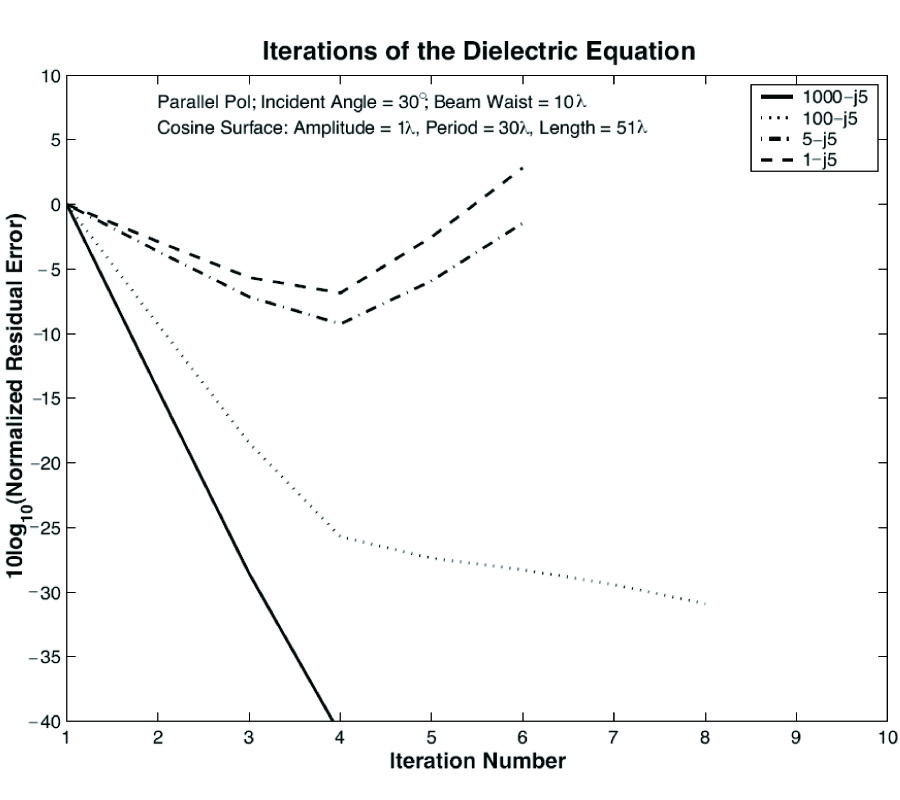An Exact Perturbative Formulation of the Dielectric Integral Equations for Lossy Interfaces

In modeling scattering from lossy surfaces, the surface is often approximated as a perfect electric conductor (PEC). However, when loss and wave penetration become important, the IBC model is typically employed and is adequate for many numerical simulations. However, the IBC's range of validity is considered unclear and an accurate quantification of its error is difficult. Consequently, other more exact implementations are necessary, such as integral equation methods. In this paper, a novel numerical implementation of the exact dielectric integral equations has been developed for scattering from a two-dimensional (2D), lossy dielectric interface. The formulation presented herein combines the coupled integral equations to form a single equation. This equation is easily interpreted as the magnetic field integral equation (MFIE) for a 2D, PEC surface with a perturbative term related to the finite conductivity of the surface. The advantage of this perturbation approach is that for ocean and other high loss surfaces, the solution is expected to be rapidly convergent with respect to other approaches and will reproduce the correct result even for surfaces with small curvature radii. Test cases demonstrate increased convergence with increased loss and increased contrast for perpendicular polarization. However with parallel polarization, convergence problems are uncovered and are associated with the Brewster angle effect.

PIER Vol. 42, 247-259, 2003. doi:10.2528/PIER03030701

###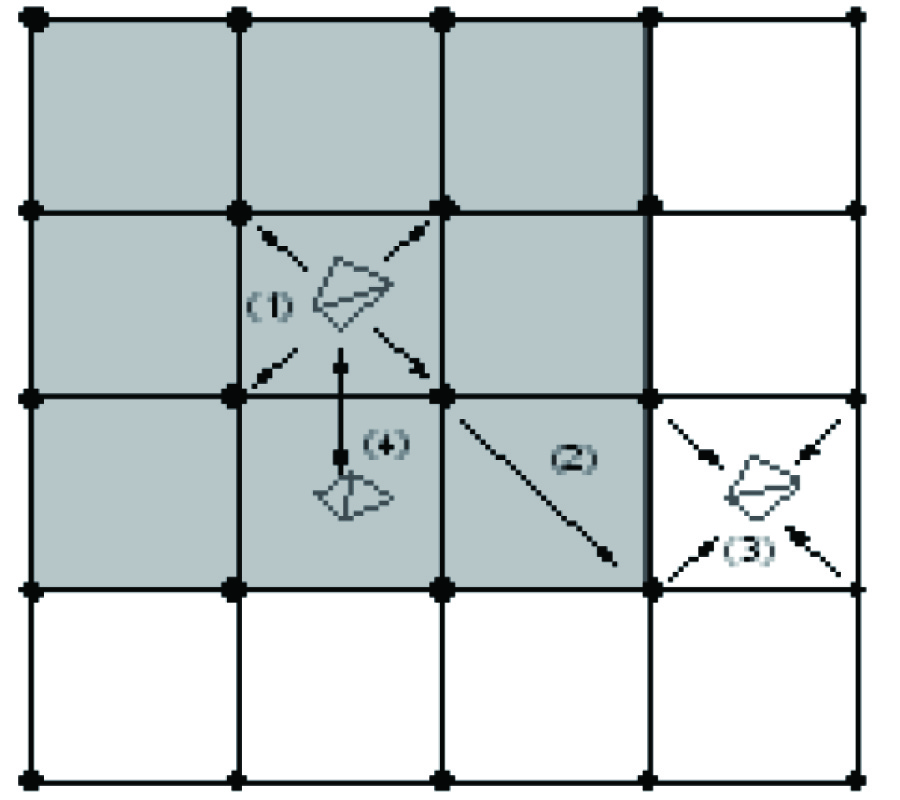Mpi-Based Parallelized Precorrected FFT Algorithm for Analyzing Scattering by Arbitrarily Shaped Three-Dimensional Objects

In this paper, how to parallelize the Pre-corrected FFT algorithm for solving the scattering problem of large scale is presented and discussed. The P-FFT technique developed by our group earlier was extended in the current analysis. To show the efficiency of the MPI-based parallelization algorithm, the experiment results are given in the latter part of the paper and various comparisons are made for such a demonstration.

PIER Vol. 42, 233-246, 2003. doi:10.2528/PIER03012101

###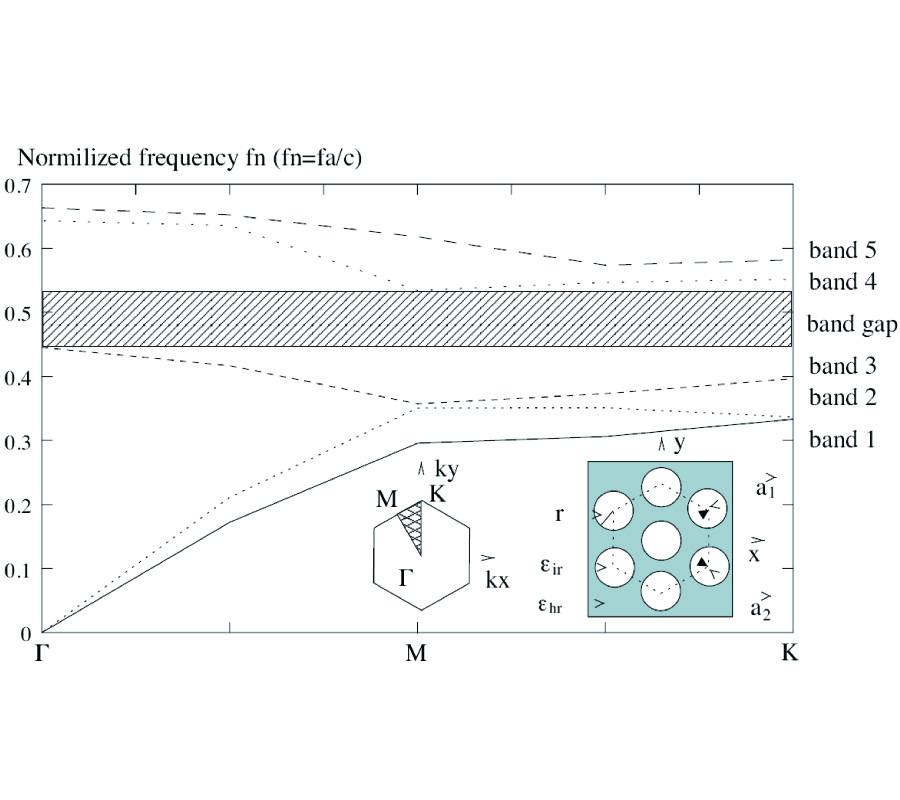Analysis of BI-Anisotropic PBG Structure Using Plane Wave Expansion Method

An algebraic eigenvalue problem for analyzing the propagation characteristics of electromagnetic waves inside the PBG Structure consisting of complex medium is established by using Bloch theorem and plane wave expansion. Two eigen-solvers are employed. One is matrix-based and another is iterative. Calculated results show that both methods are effective for 2-D PBG structure, but the iterative eigen-solver is more attractive in both CPU-time and memory requirement. A sample of 2-D PBG structure, with Chiral medium as host and air cylinders arranged in triangular lattice as inclusion, is analyzed using both methods. It is found that the introduction of chirality increases the band gap width significantly.

PIER Vol. 42, 219-232, 2003. doi:10.2528/PIER03022202

###A Coupled-Mode Theory-Based Analysis of Coupled Microstrip Lines on a Ferrite Substrate

Presented herein is a coupled-mode formulation for coupled microstrip lines on a magnetized ferrite substrate. The formulation discussed here is an extension of the coupled-mode theory for microstrip lines on an isotropic substrate. Since the magnetized ferrite exhibits a biaxial anisotropy in its permeability, the guidedwave fields in the magnetized ferrite are not subject to the conventional reciprocity relation for fields in an isotropic medium. Thus, a generalized reciprocity relation is first derived from two sets of guidedwave fields, which propagate in ferrite magnetized transversely along the strip surface. The reciprocity relation is then used to derive coupled-mode equations for coupled microstrip lines on a ferrite substrate. As a basic numerical example, the new formulation is applied to two coupled microstrip lines on a ferrite substrate.

PIER Vol. 42, 193-218, 2003. doi:10.2528/PIER03020801

###Asymptotic Radiation Field of Asymmetric Planar Dielectric Waveguide

This paper describes the steepest-descent evaluation of the radiation field for both TE and TM modes of an asymmetric planar open waveguide. The cover, film and substrate field will be formulated in the spectral domain. The steepest-descent path in the complex axial transform plane (Î¶-plane) is identified as a direct method and that in the complex Ï†-plane (Ï† = Ïƒ +jÎ·: complex polar coordinate) is also identified as an indirect method in order to validate the steepestdescent path in the complex axial transform plane (Î¶-plane). The branch cut integration will be rigorously analyzed through complexphasor diagrams. An alternative integration path will be also identified since it is an effective method to validate the steepest-descent and branch cut integrations. Then, the steepest-descent evaluation of cover and substrate fields and numerical results for TE modes will be presented and numerical implementation for TM modes will be accommodated in the future research.

PIER Vol. 42, 173-192, 2003. doi:10.2528/PIER03012303

###Wave Propagation in a Curved Waveguide with Arbitrary Dielectric Transverse Profiles

A rigorous approach is derived for the analysis of electromagnetic (EM) wave propagation in dielectric waveguides with arbitrary profiles, situated inside rectangular metal tubes, and along a curved dielectric waveguide. The first objective is to develop a mode model in order to provide a numerical tool for the calculation of the output fields for radius of curvature 0.1 m â‰¤ R â‰¤ âˆž. Therefore we take into account all the terms in the calculations, without neglecting the terms of the bending. Another objective is to demonstrate the ability of the model to solve practical problems with inhomogeneous dielectric profiles. The method is based on Fourier coefficients of the transverse dielectric profile and those of the input wave profile. These improvements contribute to the application of the model for inhomogeneous dielectric profiles with single or multiple maxima in the transverse plane. This model is useful for the analysis of dielectric waveguides in the microwave and the millimeter-wave regimes, for diffused optical waveguides in integrated optics, and for IR regimes.

PIER Vol. 42, 143-171, 2003. doi:10.2528/PIER03011701

###Eigenfunctional Representation of Dyadic Green's Functions in Cylindrically Multilayered Gyroelectric Chiral Media

This paper presents an eigenfunction expansion of the electric-type dyadic Green's functions for both a unbounded gyroelectric chiral medium and a cylindrically-multilayered gyroelectric chiral medium in terms of the cylindrical vector wave functions. The unbounded and scattering Green dyadics are formulated based on the principle of scattering superposition for the electromagnetic waves, namely, the direct wave and scattered waves. First, the unbounded dyadic Green's functions are correctly derived and some mistakes occurring in the literature are pointed out. Secondly, the scattering dyadic Green's functions are formulated and their coefficients are obtained from the boundary conditions at each interface. These coefficients are expressed in a compact form of recurrence matrices; coupling between TE and TM modes are considered and various wave modes are decomposed one from another. Finally, three cases, where the impressed current source are located in the first, the intermediate, and the last regions respectively, are taken into account in the mathematical manipulation of the coefficient recurrence matrices for the dyadic Green's functions.

PIER Vol. 42, 131-142, 2003. doi:10.2528/PIER03010602

###Measurement of Complex Permittivity of Liquids Using Waveguide Techniques

Complex permittivity of a number of liquids and binary mixtures has been studied by measurement using the waveguide techniques at the X and Ku band. Particular pieces of WR90 and WR62 waveguides were designed for the measurement of liquid materials. The custom designed TRL calibration kits are applied for calibration of the waveguide system. The measured results of complex permittivity of liquid dielectrics, such as methanol, propyl alcohol, ethyl alcohol, chlorobenzene, dioxane, cyclohexane and binary mixtures, are presented. Particular pieces of open-ended waveguides for the X and Ku bands were also designed for holding liquids and the measured data using the open-ended waveguide technique were compared with those measured using the waveguide technique. Some of the measured results are also compared with calculated data using the Debye equation and published data measured by the Fourier transform spectroscopy.

PIER Vol. 42, 107-130, 2003. doi:10.2528/PIER03012302

###Numerical Analysis of Some Scattering Problems in Continuous Random Medium

In this paper, we present numerical analysis for the following scattering problems: the radar cross-section, the backscattering enhancement, and the angular correlation function for waves scattered from practical targets embedded in random media. We assume perfect conducting targets with various cross-sections to study the effect of target configuration on the scattering problems. Also, we consider different random media with taking account of incident wave polarization. The scattering waves from conducting targets in random media can be estimated by a numerical method that solves the scattering problem as a boundary value problem. In this method, we use current generator and Green's function to obtain an expression for the scattering waves.

PIER Vol. 42, 91-105, 2003. doi:10.2528/PIER03012901

###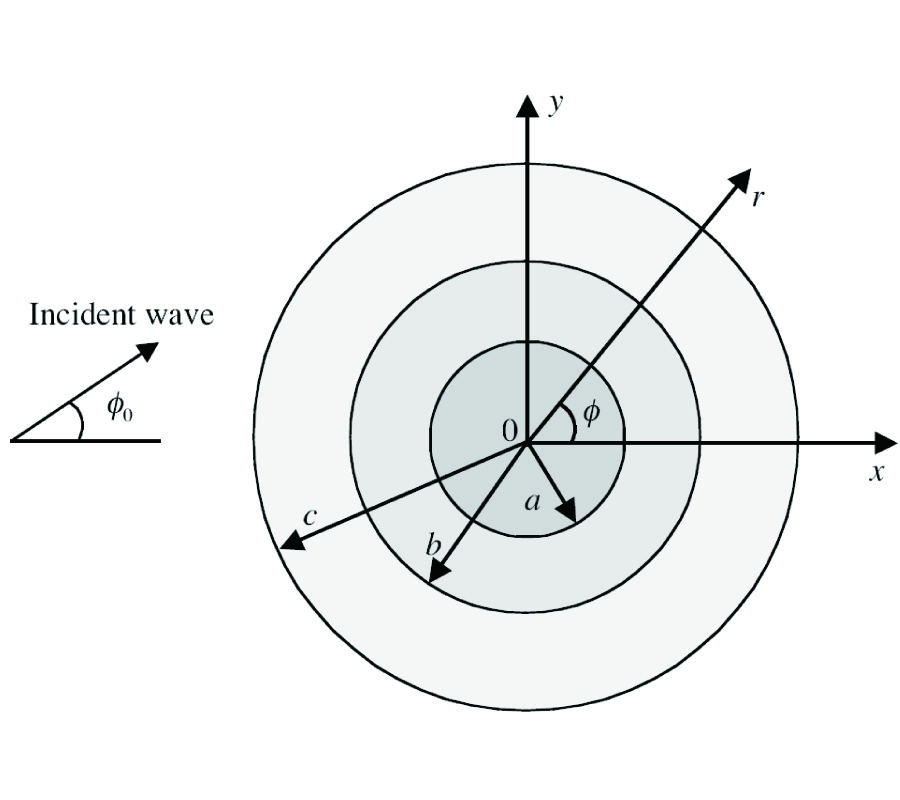Electromagnetic Scattering by a Conducting Cylinder Coated with Metamaterials

The electromagnetic scattering from a conducting cylinder coated with metamaterials, which have both negative permittivity and permeability, is derived rigorously by using the classic separation of variables technique. It is found that a conducting cylinder coated with metamaterials has anomalous scattering cross section compared to that coated with conventional dielectric materials. Numerical results are presented and discussed for the scattering cross section of a conducting cylinder coated with metamaterials.

PIER Vol. 42, 49-89, 2003. doi:10.2528/PIER03010901

###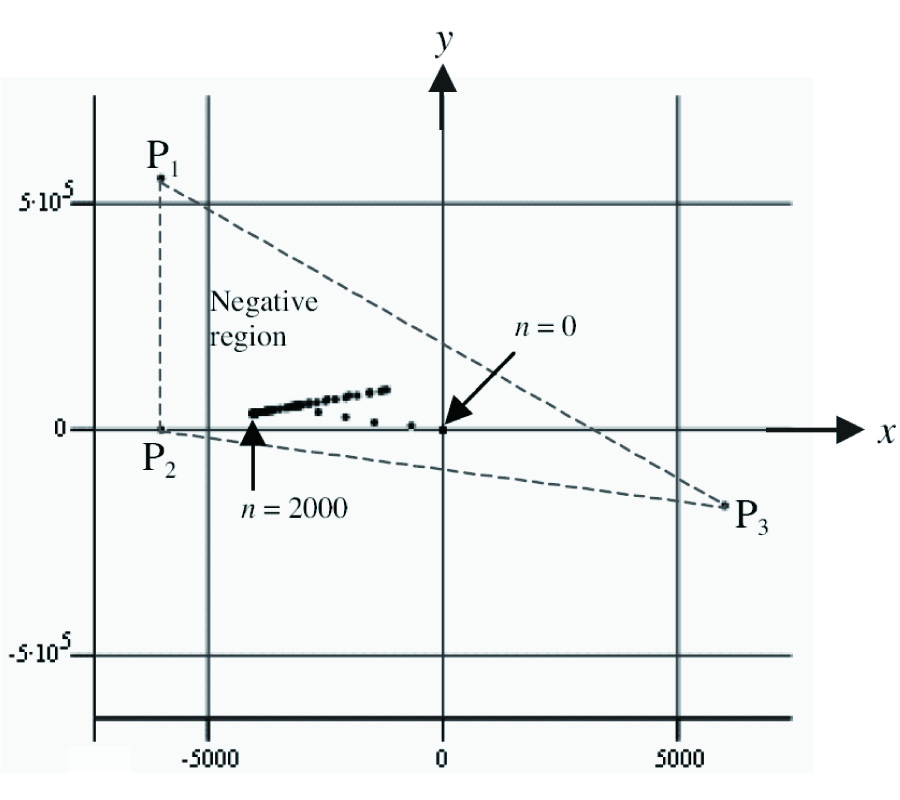Stability of Classical Finite-Difference Time-Domain (FDTD) Formulation with Nonlinear Elements --- a New Perspective

In this paper new stability theorems for Yee's Finite- Difference Time-Domain (FDTD) formulation are derived based on the energy method. A numerical energy expression is proposed. This numerical energy is dependent on the FDTD model's E and H field components. It is shown that if the numerical energy is bounded, then all the field components will also be bounded as the simulation proceeds. The theorems in this paper are inspired by similar results in nonlinear dynamical system. The new theorems are used to prove the stability of a FDTD model containing non-homogeneous dielectrics, perfect electric conductor (PEC) boundary, nonlinear dielectric and also linear/nonlinear lumped elements. The theorems are intended to complement the well-known Courant-Friedrich-Lewy (CFL) Criterion. Finally it is shown how the theorems can be used as a test, to determine if the formulation of new lumped element in FDTD is proper or not. A proper formulation will preserve the dynamical stability of the FDTD model. The finding reported in this paper will have implications in the manner stability analysis of FDTD algorithm is carried out in the future.

PIER Vol. 42, 27-48, 2003. doi:10.2528/PIER03022504

###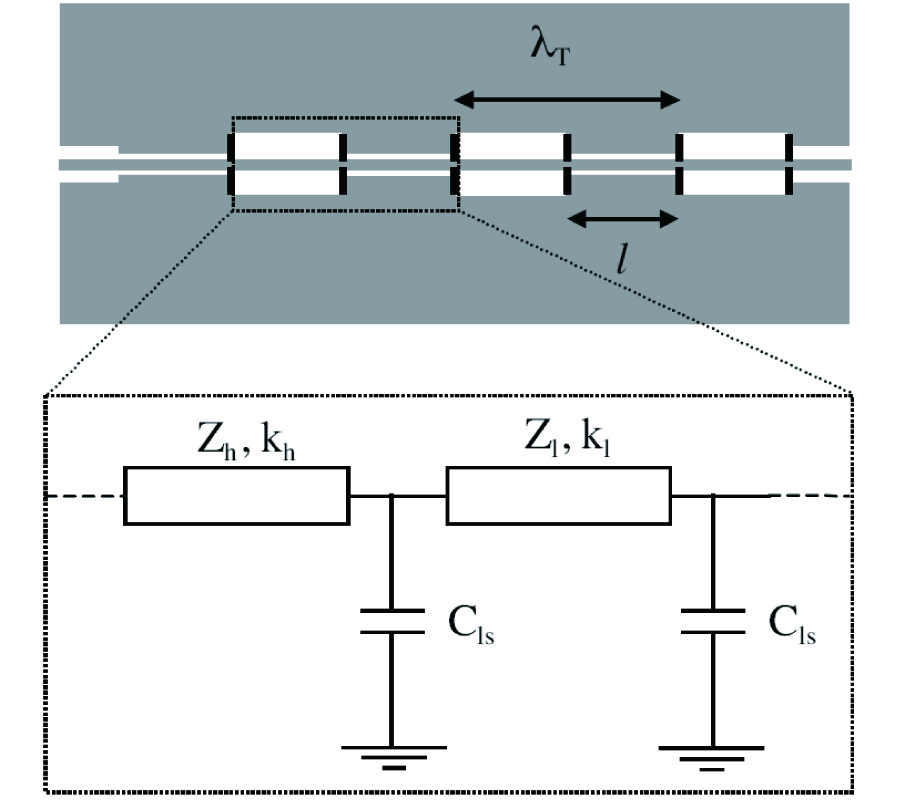Analysis of the Reflection Properties in Electromagnetic Bandgap Coplanar Waveguides Loaded with Reactive Elements

Abstract-In this work, we study the reflection properties of coplanar waveguides (CPW) periodically loaded with shunt connected capacitances and periodically perturbed by varying the slot width. These structures are of interest because the low pass frequency response with spurious frequency bands, inherent to the presence of capacitors, can be improved. This is achieved through the attenuation of frequency parasitics that is obtained by the introduction of slot width modulation. Both sinusoidal and square wave patterns are considered and the effects of the relative position of reactive elements with regard to the perturbation geometry is analysed. According to coupled mode theory, the central frequencies of the rejected bands in periodic transmission media are given by the spectrum of the perturbation function. However, it is demonstrated that, due to the presence of capacitors, multiple spurious bands can be simultaneously suppressed even in the case of a singly tuned (sinusoidal) perturbation geometry. This result points out that the frequency selective behaviour associated to the presence of slot width modulation can not be interpreted in the framework of coupled mode theory, since the rejection of spurious bands in periodic loaded CPWs is not merely given by the spectrum of the perturbation geometry.

PIER Vol. 42, 1-25, 2003. doi:10.2528/PIER03012702

###Homogenization of Spherical Inclusions

The homogenization of cubically arranged, homogeneous spherical inclusions in a background material is addressed. This is accomplished by the solution of a local problem in the unit cell. An exact series representation of the effective relative permittivity of the heterogeneous material is derived, and the functional behavior for small radii of the spheres is given. The solution is utilizing the translation properties of the solutions to the Laplace equation in spherical coordinates. A comparison with the classical mixture formulas, e.g., the Maxwell Garnett formula, the Bruggeman formula, and the Rayleigh formula, shows that all classical mixture formulas are correct to the first (dipole) order, and, moreover, that the Maxwell Garnett formula predicts several higher order terms correctly. The solution is in agreement with the Hashin-Shtrikman limits.